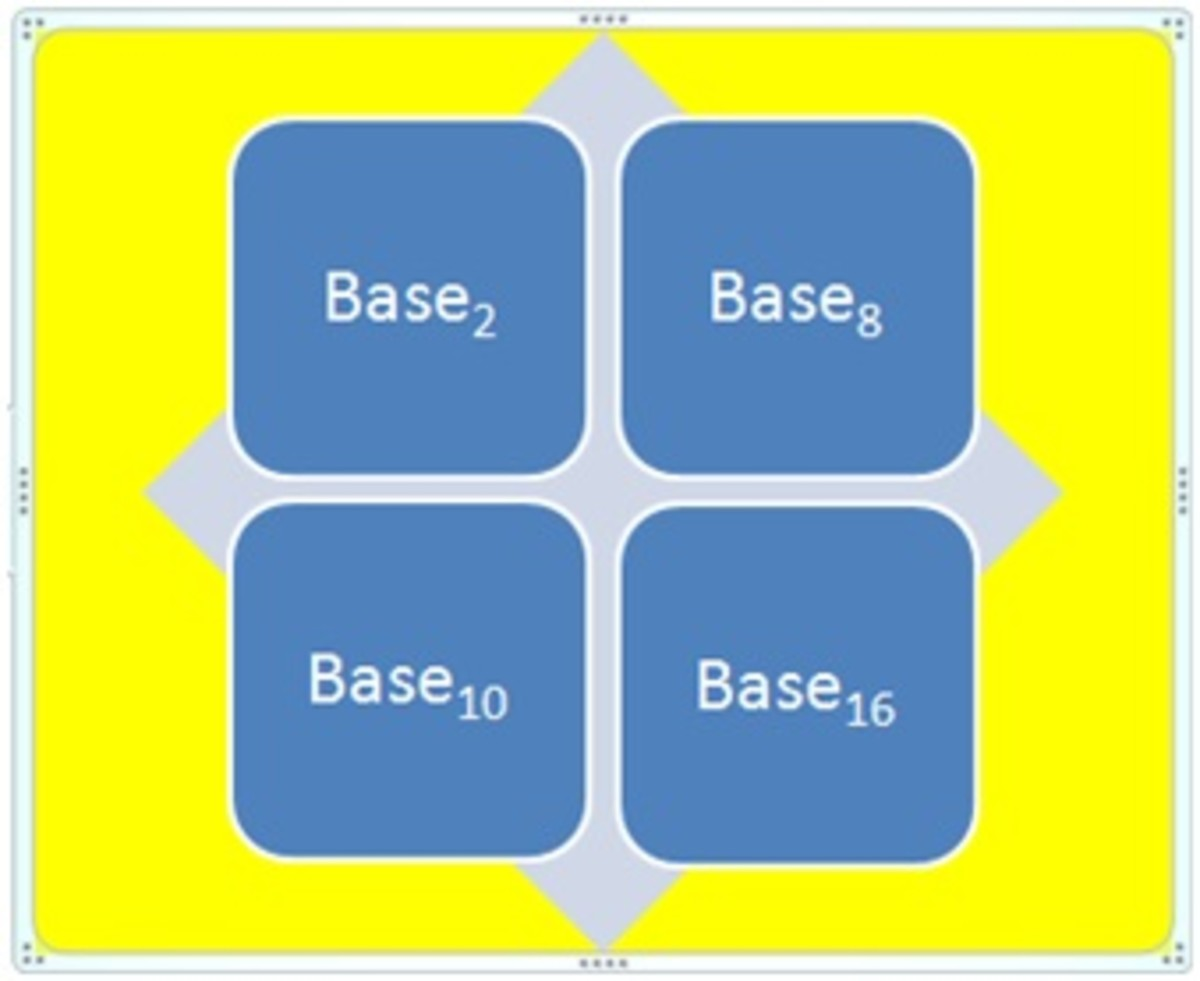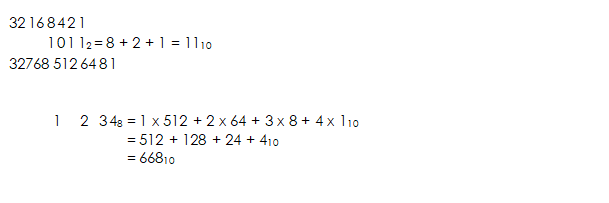# Overview of Number Bases

Welcome to class!

In today’s class, we will be talking about an overview of number bases. Enjoy the class!

### Overview of Number BasesHuman nature dictates that we try to quantify everything we come in contact with, e.g. the number of students in a class, the number of eggs in a basket, etc. If we think about the type of things humans try to quantify, we can see that they are not all quantified in the same units of measure, e.g. time is measured in hours, minutes and seconds but the distance from Glasgow to Edinburgh is measured in miles. Therefore, a number system defines a set of values used to represent a quantity.

Number Systems can be traced back to the early civilizations of Egypt and Babylon. These cultures could perform arithmetic operations on whole numbers, i.e. numbers without a decimal point. Number bases are different ways of writing and using the same number. We use a system called base 10, or denary, for our arithmetic, but there are almost as many number bases as there are numbers. Many people think that we use base 10 because we have 10 fingers on which we can count. Computers, and other electronic devices, can only reliably use an electrical current, or the absence of a current, to count (like having two fingers), and so they tend to use base 2 (binary) internally.

Many number systems have been, and still are in use, some of which may be familiar, these include Arabic, Babylonian, Mayan and Roman. The Roman number system uses numerals to represent each number, e.g. the number 5 is represented as V. In contrast, the most commonly used system is the Arabic system which uses the digits 0 to 9. This number system was used as early as the 3rd Century BC.

Each number system can be defined by its base (sometimes referred to as the radix). This base value of the number system indicates the number of different values the set has before repeating itself, e.g. Decimal has a base of ten values, hence, the digits 0-9, Octal has a base of 8 values, hence the digits 0-7.

You can work in any number base (except 1, which wouldn’t make sense), and some programming languages such as Lisp let you do that. In computing, however, you generally only come across the following four bases, and you know base 10 already. These common bases also have proper names, shown in parentheses:

• base 2 (binary)
• base 8 (octal)
• base 10 (denary)

The largest digit you can have in any column is the one less than the number of the base. So for binary (base 2), it’s 1, then 7 for Octal (base 8), 9 for Denary (base 10), etc.

After base 10, however, we run out of digits to represent the numbers, so we have to use letters, where A = 10, B = 11, C = 12, etc. So the sequence for numbers written in Hexadecimal is 0, 1, 2, 3, 4, 5, 6, 7, 8, 9, A, B, C, D, E, F, followed by 10, 11, 12, 13, 14, 15, 16, 17, 18, 19, 1A, 1B, 1C, 1D, 1E, 1F, etc.

When we write a number in base 10, we know its value because we multiply the individual digits by their corresponding column headings. For example, when we see 123, even if we don’t think about it, we calculate 1 x 100 + 2 x 10 + 3 x 1 to give us one hundred and twenty-three. Other number bases work in the same way.

You can use this information to convert numbers in other bases to base 10, e.g.:#### Binary (Base 2):

You probably won’t encounter binary much these days, but it’s useful to understand that this is how computers work internally, so you can understand concepts such as parallel transmission. The fact that computers use binary is why everything is a multiple of 2 – why computers come with 8Mb, 16Mb, 32Mb, 64Mb, etc., of memory, rather than 10Mb, 20Mb, 30Mb, etc., and also why there are 1024 bytes in a kilobyte (1024 = 210) rather than 1000 bytes.

Its main use is probably in combination with the bitwise logic techniques shown on the previous page, to combine and split values stored in the same byte (or word).

###### Evaluation
1. (1010.011)2 = (a) (10.365)10   (b) (10.375)10   (c) (11.365)10    (d) (11.375)10
2. (41)⊂10 in binary is  (a) 101101   (b) 101011  (c) 101001  (d) 101101
3. Convert (0.6875)⊂10 to binary  (a) 0.1011  (b) 0.1011  (c) 0.0101  (d) 0.0111
4. Convert (153.513)10 in octal number system is(a) 231.408517  (b) 231.407517  (c) 231.406517  (d) 231.406617
5. All these are different types of number systems except  (a) Arabic  (b) Babylonian  (c) Roman  (d) Nigerian

In our next class, we will be talking more about Overview of Number Bases.  We hope you enjoyed the class.

Should you have any further question, feel free to ask in the comment section below and trust us to respond as soon as possible.

How Can We Make ClassNotesNG Better - CLICK to Tell Us💃